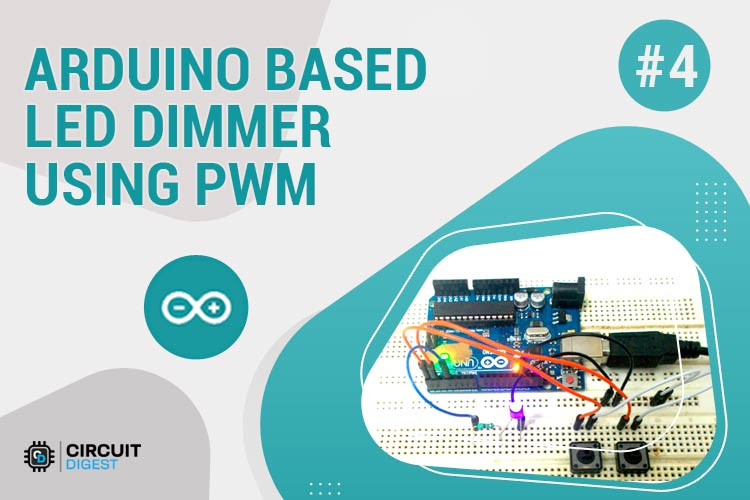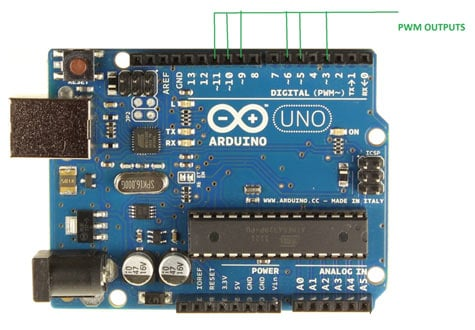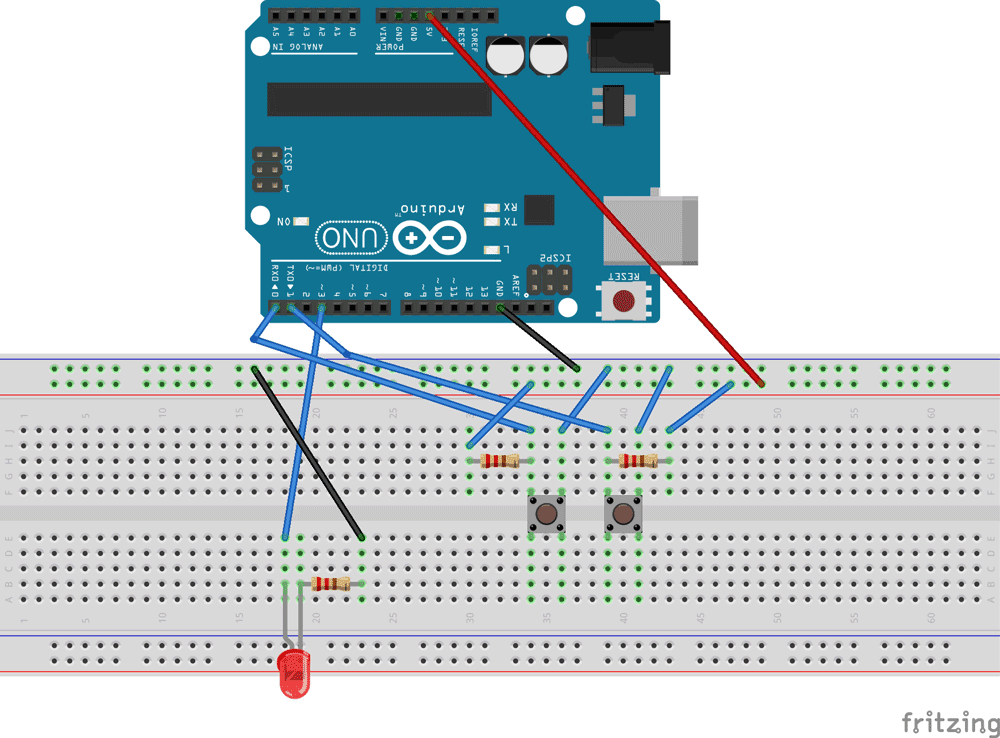# Arduino Based LED Dimmer using PWM

Published  August 6, 2015   8This LED DIMMER is an Arduino Uno based PWM (Pulse Width Modulation) circuit developed to get variable voltage over constant voltage. The method of PWM is explained below. Before we get start building a 1 Watt LED Dimmer circuit, first consider a simple circuit as shown in figure below.Now if the switch in the figure is closed continuously over a period of time then the bulb will continuously ON during that time. If the switch is closed for 8ms and opened for 2ms over a cycle of 10ms, then the bulb will be ON only in the 8ms time. Now the average terminal over across the over a period of 10ms = Turn ON time/ (Turn ON time + Turn OFF time), this is called duty cycle and is of 80% (8/ (8+2)), so the average output voltage will be 80% of the battery voltage.

In the second case, the switch is closed for 5ms and opened for 5ms over a period of 10ms, so the average terminal voltage at the output will be 50% of the battery voltage. Say if the battery voltage is 5V and the duty cycle is 50% and so the average terminal voltage will be 2.5V.

In the third case the duty cycle is 20% and the average terminal voltage is 20% of the battery voltage.

Now how this technique is used in this LED Dimmer? It is explained in the subsequent section of this tutorial.As shown in figure, an Arduino UNO has 6PWM channels, so we can get PWM (variable voltage) at any of these six pins. In this chapter we are going to use PIN3 as PWM output.

### Required Components

Hardware: ARDUINO UNO, power supply (5v), 100uF capacitor ,LED, buttons (two pieces), 10KΩ resistor (two pieces).

Software: arduino IDE

### Circuit Diagram and ExplanationThe circuit is connected on breadboard as per the circuit diagram. However one must pay attention during connecting the LED terminals. Although the buttons show bouncing effect in this case it does not cause considerable errors so we need not worry this time.

The PWM from UNO is quite easy. While setting up a ATMEGA controller for PWM signal is not easy, we have to define many registers and settings for a accurate signal, however in ARDUINO we don’t have to deal with all those things.

By default all the header files and registers are predefined by ARDUINO IDE, we simply need to call them and that’s it we will have a PWM output at appropriate pin.

Now for getting a PWM output at a appropriate pin, we need to work on two things,

 pinMode(ledPin, OUTPUT) analogWrite(pin, value)

First we need to choose the PWM output pin from six pins, after that we need to set that pin as output.

Next we need to enable the PWM feature of UNO by calling the function “analogWrite(pin, value)” . Here ‘pin’ represent the pin number where we need PWM output we are putting it as ‘3’. So at PIN3 we are getting PWM output. Value is the turn ON duty cycle, between 0 (always off) and 255 (always on). We are going to increment and decrement this number by button press.

Using PWM pins in Arduino Uno is explained in the C code given below.

Code

volatile int i=0;//initializing a integer for incrementing and decrementing duty ratio.

void setup()

{

pinMode(3, OUTPUT);   // sets the pin3 as output

pinMode(0, INPUT);// sets the pin0 as output

pinMode(1, INPUT);// sets the pin1 as output

}

void loop()

{

analogWrite(3, i);  // analogWrite values from 0 to 255

{

if (i<255)

{

i++;//if pin0 is pressed and the duty ratio value is less than 255

delay(30);

}

}

{

if (i>0)

{

i--;// if pin1 is pressed and the duty ratio value is greater than 0

delay(30);

}

}

}

Video

Tags

Submitted by Henk Don on Sat, 03/19/2016 - 21:36

### Code

De code pinMode 0 and 1 are nor working.
I modyfi pinMode(4, INPUT), and pinMode(7, INPUT)
and it's working
pin 0=rx and pin =tx.
Kind regards;
Henk Don
the Nederlands

### add the lcd display module

If i want  attach the frequency counter program with this program so what should i do?

Submitted by Iqbal on Thu, 07/06/2017 - 13:42

### motor speed control

hello, great project, i have done this and it is working, how can we add ac 200Watt motor for speed control with this same circuit by 2 switch , 1 for speed up and other for speed down. same as this circuit?

Submitted by Mushtabah on Fri, 09/29/2017 - 23:51

### if i want to make that

if i want to make that project but control by web page..so what should i do ?

Submitted by RAJNIK makani on Sat, 02/03/2018 - 12:45

### Can I control ac fan using

Can I control ac fan using this program

Submitted by RUPALI SUDAM K… on Sun, 03/11/2018 - 08:25